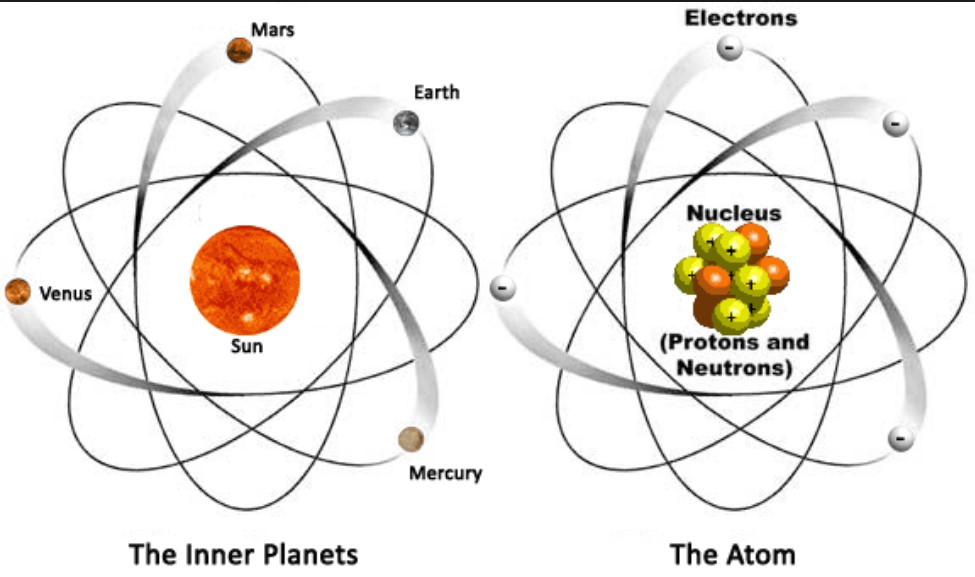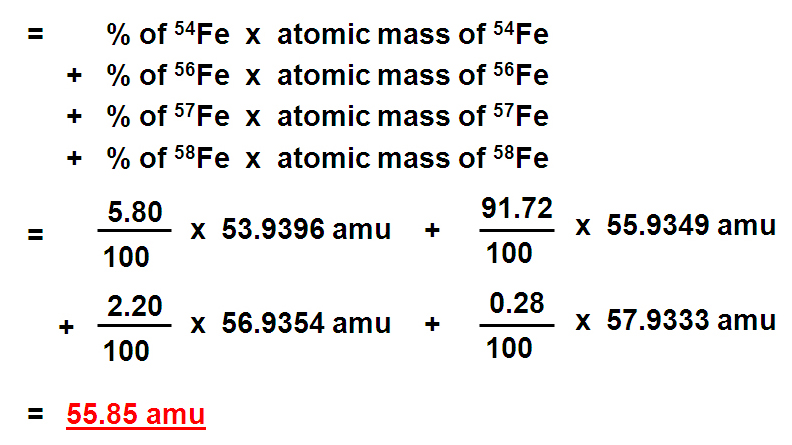# Atomic Theory and Structure

What follows here is the supporting content for lecture 4 of general chemistry I. This lecture will cover the History of Development of the atomic theory, atomic structure, chemical symbolism and calculations of atomic mass.

We will start with a review of the steps taken to create the atomic theory:

Now that we have reviewed how we got to the Atomic Theory, let's look at the atomic structure we now believe to be true.

Atomic Structure is fairly simple. It is often described as similar to the solar system with the nucleus representing the sun and the electrons acting as the planets:The atom is composed of three types of particles located in two areas. The protons and neutrons are located in the nucleus and the electrons are located in energy levels surrounding the nucleus.Now that you are familiar with the particles that make up each atom in an element, let's go through how these particles are represented in the periodic table. In other words, the information that is given in the symbolism used in the periodic table that allows you to determine the protons, neutrons and electrons contained in each atom or element.

We can represent an element or the atom of an element by its symbol.

• C = carbon
• O = oxygen
• B = boron

We also use these symbols to show how atoms (in molecules) change partners in a chemical reaction.

• CO + PbO → CO2 + Pb
• NH3 + HCl → NH4Cl

How do the elements themselves exist?

Monatomic elements

He, Ne, Ar, Kr, Xe, Rn
(called Noble Gases, they used to be called the inert gases)

Diatomic elements

H2, O2, N2, F2, Cl2, Br2, I2
(Oxygen also exists as O3 = ozone. This is called an allotropic form; many elements exist in several allotropic forms.)

Other forms of elements

P4, S8, C60, etc
Diamond, graphite, and buckminsterfullerene ("buckyball," C60) are all forms of the same element, carbon.

In the periodic table, each element is given a box containing its chemical symbol as well as several other pieces of information:Looking at the symbol for Magnesium you will notice a few numbers: 24, 12 and 2+. Each of these numbers provides a clue to the atomic structure of this particular magnesium atom.

Let's start with the most important of the three: The number 12. This is called the Atomic Number and correlates to the number of Protons in the atom. The number of protons is unique to each element so if you change the number of protons you change the element. In other words, EVERY atom of Magnesium will have exactly 12 protons in its nucleus.

The next number to look at is the 24. This is called the Atomic Mass Number and is a rounded value of the atomic mass of the atom. This mass is determined by adding up all of the masses of the protons and neutrons in the nucleus. So if you know the atomic Mass Number and you know the atomic number you can easily calculate the number of neutrons in the atom:

## 24 (protons and neutrons) - 12 protons= 12 Neutrons

The last number on the symbol can be used to calculate the number of electrons in the element or ion if there is a + or - charge. We know that protons carry a +1 charge and electrons carry a -1 charge from atomic theory. So if there are an equal number of protons and electrons present in the atom, the overall charge is 0 or neutral.

## Protons + Electrons = Charge or Ionization State

So if there is a charge shown as in the example above, 2+, this means there are two more protons than there are electrons and

## (12+) - (?e-) = 2+ thus the number of electrons is 10.

Let's Practice:

Finally, we need to discuss the atomic mass number and then the atomic mass more thoroughly.

### Isotopes

Since we need to calculate the number of neutrons present in an atom from the atomic mass number this implies that there is more than one atomic mass number. If there was only one like in the case of the atomic mass, we would not need a calculation to determine the number of neutrons, it would just be known, right? So since we can calculate the number of neutrons present in each atom, this means that even atoms of the same element can have differing numbers of neutrons and this is in fact true. We call atoms of elements with different numbers of neutron Isotopes.

There are many known isotopes for each element with very few exceptions only having one. You can locate those elements with only one isotope by looking at their atomic mass shown in the periodic table. Can you guess how? Well, let me give you a hint. The atomic mass listed in the periodic table for each element is calculated by taking the "percent abundance" of each Isotope and multiplying that by its mass. So basically this is a weighted average mass. BUT if there was only one isotope, there would only be one mass, right? So this would be how you could figure out which element(s) only have one isotope.

### Atomic Mass

So I used a couple of terms above that I have found causes so mathematical panic in students previously so let me give an example that might make the "weighted average atomic mass" and its use of "percent abundance" more understandable.

Suppose you wanted to calculate the average mass of a group of 25 people. 13 of the people are women and the other 12 are men (duh). Since most men weigh more than most women, assigning a single weight to everyone would not make for a very accurate average weight. But if each man weighed ~180lbs and each woman weighed ~130lbs you could take the % of each (Women 13/25 = 0.52 or 52% and Men 12/25 = 0.48 or 48%) and multiply those percentages by the masses and get pretty close to the average mass of the group.

So (0.52 x 130lbs) + (0.48 x 180lbs) = 154lbs

This is exactly what we do to get the weighted average atomic mass. We take the percentage of each isotope and multiply that by the isotope's mass to get its individual contribution to the overall mass of the element:

Example:Here are some practice problems:

Back to HOME

Back to Segment 1